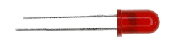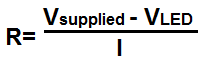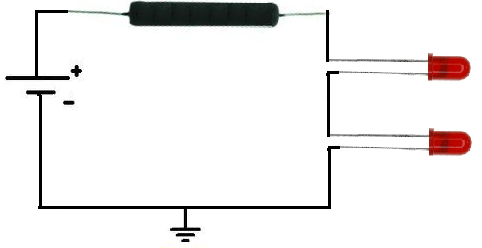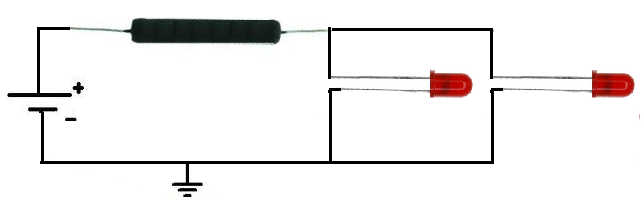﻿ LED Resistor Calculator ﻿# LED Resistor Calculator### Single LED Resistor CalculatorEnter the Supply Voltage, VS: V Enter the Forward Voltage, VF: V Enter the Desired Current, I: mA (milliamperes)

nan Ω

This LED resistor calculator calculates the value of the resistor which you would need in order to produce the desired current to go through the LED.

The factors that determine the resistance value of the resistor are the supply voltage, VS, the forward voltage drop needed for the LED, VF, and the desired current wanted to pass through the LED. All these factors determine the value of the resistor, based on ohm's law formula, R= V/I. We calculate the resistance value by calculating the total voltage, VS - VF. Once we have this value, we just divided by the desired current.

The supply voltage is the total amount of voltage which is supplied to a circuit. It is the power source of the circuit. Note that the voltage that the power source is supplying to the circuit must be greater than the forward voltage needed by the LED. So, for example, if an LED needs 3 volts dropped across its terminals, the supply voltage must be greater than 3 volts. Otherwise, the LED will not consume sufficient voltage needed to turn it on. If you enter in a LED forward voltage drop which is greater than the supply voltage, an error message will come up stating this is invalid, for the reason stated in this paragraph.

The LED forward voltage is the voltage which the LED consumes. Every LED needs a certain amount of voltage dropped across its terminal in order to function and turn on in a circuit. Typically, it can be around 2-3 volts. However, it varies. The best way to know how much voltage is needed for a particular LED is to check the datasheet for the LED in use. The datasheet of LEDs have this forward voltage value rating.

For example, in a datasheet, it may look like this:So, the LED above can use between 3V and 3.6V of voltage. However, the most common value is midway between this, 3.3V. So 3.3V is the forward voltage drop. Therefore, in a circuit, this LED will consume 3.3V. Therefore, it needs a supply voltage greater than this value.

The last factor is the desired current. The desired current is the current which you want to pass through the LED. If you have no idea how much current the LED needs, again check the datasheet. There is a rating called the forward current, IF. This is the maximum current which an LED can handle passing through it.

This is how it may look on a datasheet:Again, this is the maximum current an LED can handle, so you either want the desired current to be this value or a little lower.

Most datasheets even have a suggestion current, ISU about which value to use. This is how it may look on a datasheet:This is a good desired current value to use. What you choose depends on how bright you want the LED to shine. The more current an LED gets, the brighter it shines. The less current it receives, the more it dims. How bright or dim you want it to shine also determines what desired current value you choose. If you want it dim, you would choose the current value on the lower end of this scale. If you want it bright, you would choose the current value on the higher end of this scale.

The units for the supply voltage and forward LED voltage drop are in unit volts (V). The unit for the desired current is in milliamperes (mA).

To use this calculator, a user just has to enter in the supply voltage, VS, the LED forward voltage drop, VF, and the desired current, I, and then click the 'Submit' button, and the resultant answer will automatically be computed and shown. The resultant answer, the resistor value, will be calculated in unit ohms (Ω).

Example Calculation

If the supply voltage is 5V and the LED has a forward voltage drop of 2V and a desired current of 15mA is wanted to flow across the LED, what resistor value is best for this circuit?

So based on the values of the parameters, we use the equation R= (VSUPPLY - VForward)/I and substitute in the values into the equation.

R= (5V - 2V)/15mA= 200Ω

### Series LEDs Resistor Calculator

This is the LED resistor calculator for LEDs in series.Enter the Supply Voltage, VS: V Enter the Forward Voltage, VF: V Enter the Desired Current, I: mA (milliamperes) How many LEDs in series?:

nan Ω

This series LED resistor calculator calculates the resistor value that is needed when LEDs are placed in series.

When LEDs are in series, each LED requires its own forward voltage drop. This means that if there are 3 LEDs in series and each LED requires a 3V voltage drop, the LEDs will consume 9V of power. So the total voltage supplying the circuit must be greater than 9V.

To use this calculator, a user just enters the supply voltage, VS of the circuit, the forward voltage drop of the LEDs, VF, the desired current, I, that is wanted to be passed through the LEDs, and the number of LEDs connected in series. The user then clicks the 'Calculate' button and the resultant value, the resistance, will be computed in unit ohms (Ω).

### Parallel LEDs Resistor Calculator

This is the LED resistor calculator for LEDs in parallel.Enter the Supply Voltage, VS: V Enter the Forward Voltage, VF: V Enter the Desired Current, I: mA (milliamperes) How many LEDs in parallel?:

nan Ω

This parallel LED resistor calculator calculates the resistor value that is needed when LEDs are connected in parallel.

When LEDs are in parallel, all of the LEDs receive the same voltage across them. Therefore, each LED does not need its own voltage across it. Voltage is common to all LEDs.

However, current gets divided up in a parallel circuit, so that more current is consumed than in series. Therefore, the more LEDs that are placed in parallel, the more current is drawn and consumed from the power source.

To use this calculator, a user just enters the supply voltage, VS of the circuit, the forward voltage drop of the LEDs, VF, the desired current, I, that is wanted to be passed through the LEDs, and the number of LEDs connected in parallel. The user then clicks the 'Calculate' button and the resultant value, the resistance, will be computed in unit ohms (Ω).

Related Resources

﻿